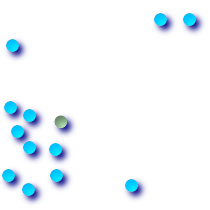## Name

ST_Centroid — Returns the geometric center of a geometry.

## Synopsis

`geometry ST_Centroid(`geometry g1`)`;

## Description

Computes the geometric center of a geometry, or equivalently, the center of mass of the geometry as a `POINT`. For [`MULTI`]`POINT`s, this is computed as the arithmetric mean of the input coordinates. For [`MULTI`]`LINESTRING`s, this is computed as the weighted length of each line segment. For [`MULTI`]`POLYGON`s, "weight" is thought in terms of area. If an empty geometry is supplied, an empty `GEOMETRYCOLLECTION` is returned. If `NULL` is supplied, `NULL` is returned.

The centroid is equal to the centroid of the set of component Geometries of highest dimension (since the lower-dimension geometries contribute zero "weight" to the centroid).Computation will be more accurate if performed by the GEOS module (enabled at compile time).This method implements the OpenGIS Simple Features Implementation Specification for SQL 1.1.This method implements the SQL/MM specification. SQL-MM 3: 8.1.4, 9.5.5

## Examples

In each of the following illustrations, the blue dot represents the centroid of the source geometry.Centroid of a `MULTIPOINT`Centroid of a `LINESTRING`Centroid of a `POLYGON`Centroid of a `GEOMETRYCOLLECTION`
```SELECT ST_AsText(ST_Centroid('MULTIPOINT ( -1 0, -1 2, -1 3, -1 4, -1 7, 0 1, 0 3, 1 1, 2 0, 6 0, 7 8, 9 8, 10 6 )'));
st_astext
------------------------------------------
POINT(2.30769230769231 3.30769230769231)
(1 row)```